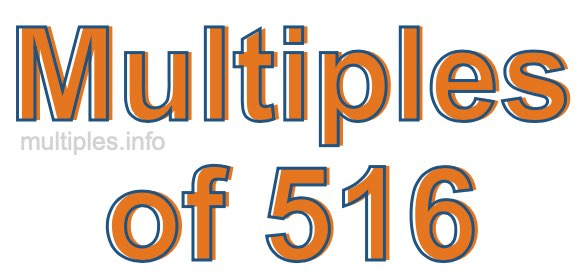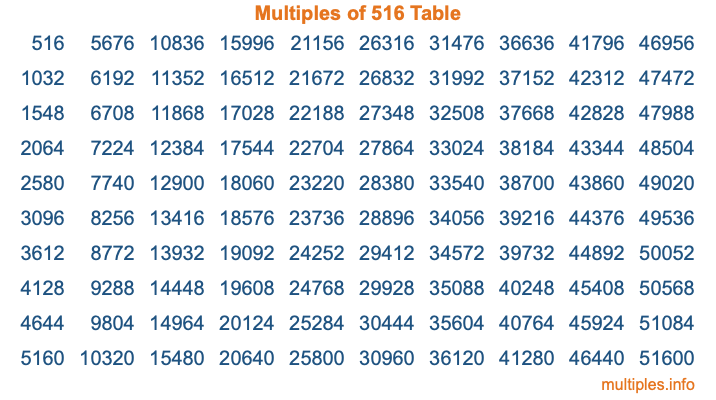Multiples of 516Welcome to the Multiples of 516 page. Here we will first teach you everything you will ever need to know about the multiples of 516, and then give you a study guide summary of everything we taught you to make sure you remember it all. Use this page to look up facts and learn information about the multiples of 516. This page will make you a multiples of five hundred sixteen expert!

Definition of Multiples of 516
Multiples of 516 are all the numbers that when divided by 516 equal an integer. Each of the multiples of 516 are called a multiple. A multiple of 516 is created by multiplying 516 by an integer.

Therefore, to create a list of multiples of 516, you start with 1 multiplied by 516, then 2 multiplied by 516, then 3 multiplied by 516, and so on for as long as you want. Thus, the list of the first five multiples of 516 is 516, 1032, 1548, 2064, and 2580. To see a larger list of multiples of 516, see the printable image of Multiples of 516 further down on this page. We also have a category where you can choose any nth multiple of 516.

Multiples of 516 Checker
The Multiples of 516 Checker below checks to see if any number of your choice is a multiple of 516. In other words, it checks to see if there is any number (integer) that when multiplied by 516 will equal your number. To do that, we divide your number by 516. If the the quotient is an integer, then your number is a multiple of 516.

Is  a multiple of 516?

Least Common Multiple of 516 and ...
A Least Common Multiple (LCM) is the lowest multiple that two or more numbers have in common. This is also called the smallest common multiple or lowest common multiple and is useful to know when you are adding our subtracting fractions. Enter one or more numbers below (516 is already entered) to find the LCM.

Check out our LCM Calculator if you need more details about the Least Common Multiple or if you need the LCM for different numbers for adding and subtraction fractions.

nth Multiple of 516
As we stated above, 516 is the first multiple of 516, 1032 is the second multiple of 516, 1548 is the third multiple of 516, and so on. Enter a number below to find the nth multiple of 516.

th multiple of 516

Multiples of 516 vs Factors of 516
516 is a multiple of 516 and a factor of 516, but that is where the similarities end. All postive multiples of 516 are 516 or greater than 516. All positive factors of 516 are 516 or less than 516.

Below is the beginning list of multiples of 516 and the factors of 516 so you can compare:

Multiples of 516: 516, 1032, 1548, 2064, 2580, etc.

Factors of 516: 1, 2, 3, 4, 6, 12, 43, 86, 129, 172, 258, 516

As you can see, the multiples of 516 are all the numbers that you can divide by 516 to get a whole number. The factors of 516, on the other hand, are all the whole numbers that you can multiply by another whole number to get 516.

It's also interesting to note that if a number (x) is a factor of 516, then 516 will also be a multiple of that number (x).

Multiples of 516 vs Divisors of 516
The divisors of 516 are all the integers that 516 can be divided by evenly. Below is a list of the divisors of 516.

Divisors of 516: 1, 2, 3, 4, 6, 12, 43, 86, 129, 172, 258, 516

The interesting thing to note here is that if you take any multiple of 516 and divide it by a divisor of 516, you will see that the quotient is an integer.

Multiples of 516 Table
Below is an image of the first 100 multiples of 516 in a table. The table is in chronological order, column by column. The first column has the first ten multiples of 516, the second column has the next ten multiples of 516, and so on.The Multiples of 516 Table is also referred to as the 516 Times Table or Times Table of 516. You are welcome to print out our table for your studies.

Negative Multiples of 516
Although not often discussed or needed in math, it is worth mentioning that you can make a list of negative multiples of 516 by multiplying 516 by -1, then by -2, then by -3, and so on, to get the following list of negative multiples of 516:

-516, -1032, -1548, -2064, -2580, etc.

Multiples of 516 Summary
Below is a summary of important Multiples of 516 facts that we have discussed on this page. To retain the knowledge on this page, we recommend that you read through the summary and explain to yourself or a study partner why they hold true.

There are an infinite number of multiples of 516.

A multiple of 516 divided by 516 will equal a whole number.

516 divided by a factor of 516 equals a divisor of 516.

The nth multiple of 516 is n times 516.

The largest factor of 516 is equal to the first positive multiple of 516.

516 is a multiple of every factor of 516.

516 is a multiple of 516.

A multiple of 516 divided by a divisor of 516 equals an integer.

516 divided by a divisor of 516 equals a factor of 516.

Any integer times 516 will equal a multiple of 516.

Multiples of a Number
Here you can get the multiples of another number, all with the same attention to detail as we did for multiples of 516 on this page.

Multiples of
Multiples of 517
Did you find our page about multiples of five hundred sixteen educational? Do you want more knowledge? Check out the multiples of the next number on our list!